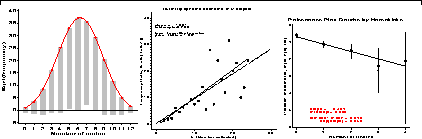# Chapter 2. Fitting and graphing discrete distributionsDiscrete data often follow various theoretical probability models. Graphic displays are used to visualize goodness of fit, to diagnose an appropriate model, and determine the impact of individual observations on estimated parameters. 2.1. Introduction 2.2. Discrete distributions 2.2.1. The binomial distribution 2.2.2. The Poisson distribution 2.2.3. The negative binomial distribution 2.2.4. The geometric distribution 2.2.5. The logarithmic series distribution 2.2.6. Power series family 2.3. Fitting discrete distributions 2.3.1. The GOODFIT macro 2.3.2. Plots of observed and fitted frequencies 2.3.3. The ROOTGRAM macro 2.3.4. Maximum likelihood estimation 2.3.5. Fitting discrete distributions as loglinear models 2.4. Diagnosing discrete distributions: Ord plots 2.5. Poissonness plot 2.5.1. Features of the Poissonness plot 2.5.2. Plot construction 2.5.3. POISPLOT macro 2.5.4. Leverage and influence 2.5.5. Plots for other distributions 2.6. Chapter summary